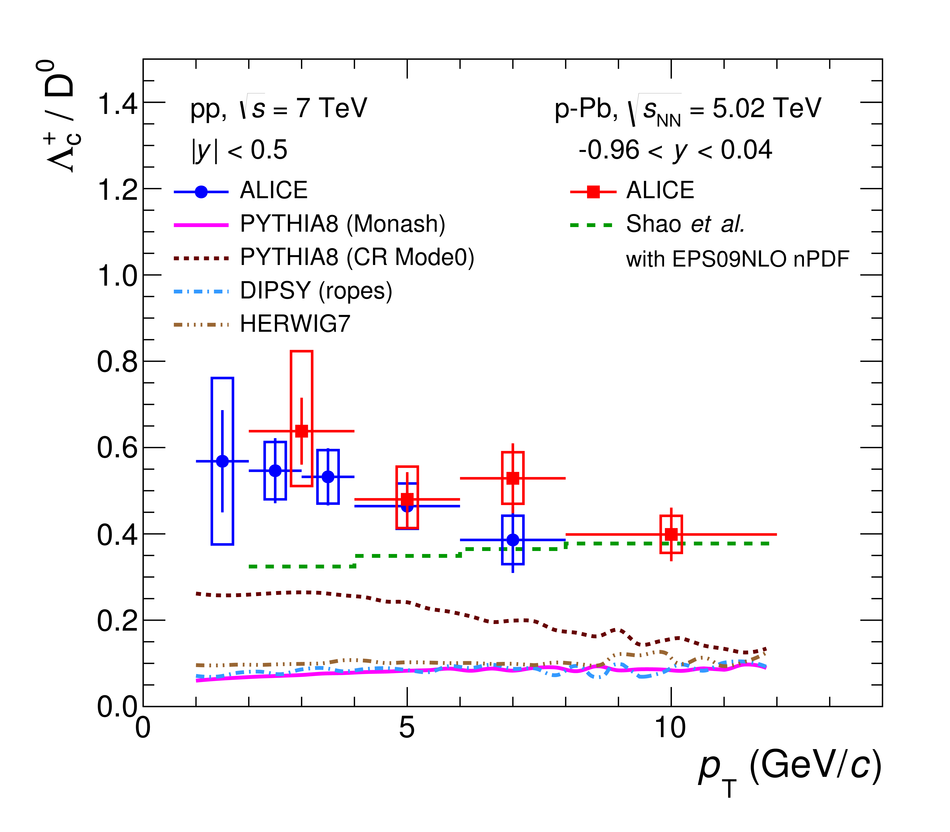# Figure 11

 The \Lcplus/\DZero ratio measured in \pp and \pPb collisions by ALICE as a function of \pt (left) and as a function of $y$ for $2 < \pt < 8$ \gevc (right). The measurements from \pp collisions are compared with different event generators (quoted tunes for {\sc pythia} and {\sc dipsy} taken respectively from~ and~). The \pPb measurement as a function of \pT is also compared with calculations from Lansberg and Shao~. The predictions from event generators as a function of $y$ are also compared with the LHCb measurement~.